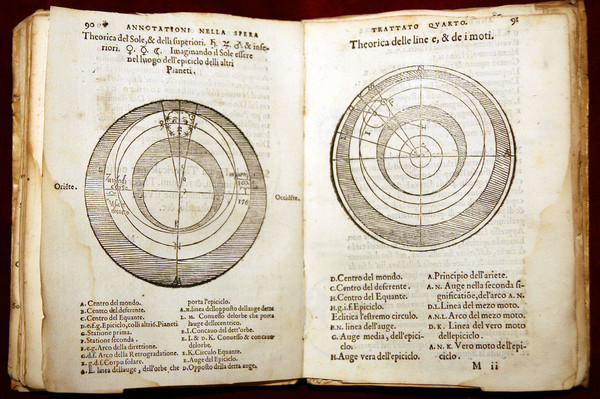# Shape of my Heart

 An animation made for this modification of ``Shape of my heart" song. There is quite a bit of Math involved in this. The math was explained and created this blog of Michael Trott which is also based on the article of Richard Clark on "Mathematics as an Art Form". So, how is the animation done? The computer algebra system takes a picture like here the picture of a heart, uses built in basic computer vision algorithm to detect the boundary curve, then makes a Fourier series of that curve, meaning that the parametrizations r(t) = [x(t),y(t)] is written as sums of sines and cosines. One can then draw the curve as generalized epicycles. When humans tried to understand the motion of the heavens, they first assumed that the planets move on circles (pure figures). When this failed, they tried to place circles on circles which is the epicycle picture of the Ptolemaic model. Further modifications were needed, one had to place circles on circles on circles etc. In principle, what the astronomers did to model the solar system was to make Fourier approximation of the actual orbit. Until Kepler figured out that the motion is an ellipse. Later, with Newton, one realized the full complexity of the system. It is an n-body problem in which all bodies interact. Also the ellipse picture is an approximation only.Source.

Direct Media Links: Webm, Ogg Ipod